# IntegersPage 1

#### WATCH ALL SLIDES

Slide 1Integers

Slide 2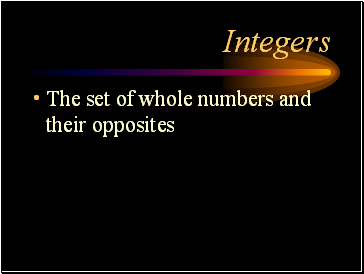## Integers

The set of whole numbers and their opposites

Slide 3## Operations with Integers

Slide 4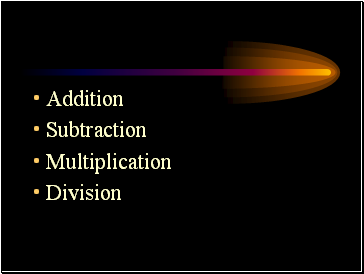Subtraction

Multiplication

Division

Slide 5Slide 6When the signs of the numbers being added are the same, add their absolute values--The sign of the answer is the same as the sign of the numbers added together

When the signs of the numbers being added are different, subtract their absolute values--The sign of the answer is the same as the number with the largest absolute value

Slide 7## Subtraction of Integers

Slide 8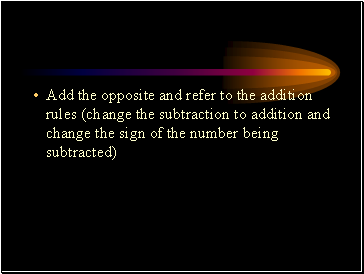Add the opposite and refer to the addition rules (change the subtraction to addition and change the sign of the number being subtracted)

Slide 9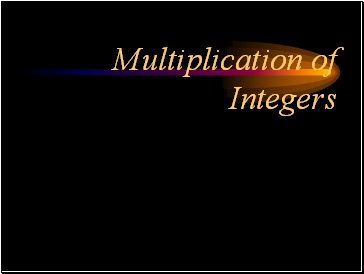## Multiplication of Integers

Slide 10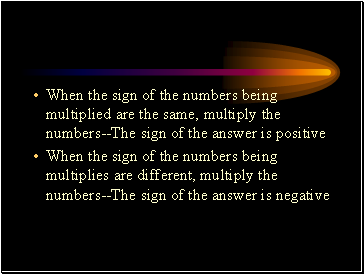When the sign of the numbers being multiplied are the same, multiply the numbers--The sign of the answer is positive

When the sign of the numbers being multiplies are different, multiply the numbers--The sign of the answer is negative

Slide 11## Division of Integers

Slide 12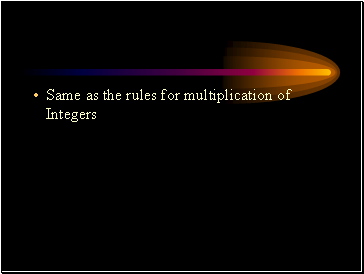Same as the rules for multiplication of Integers